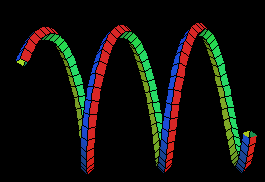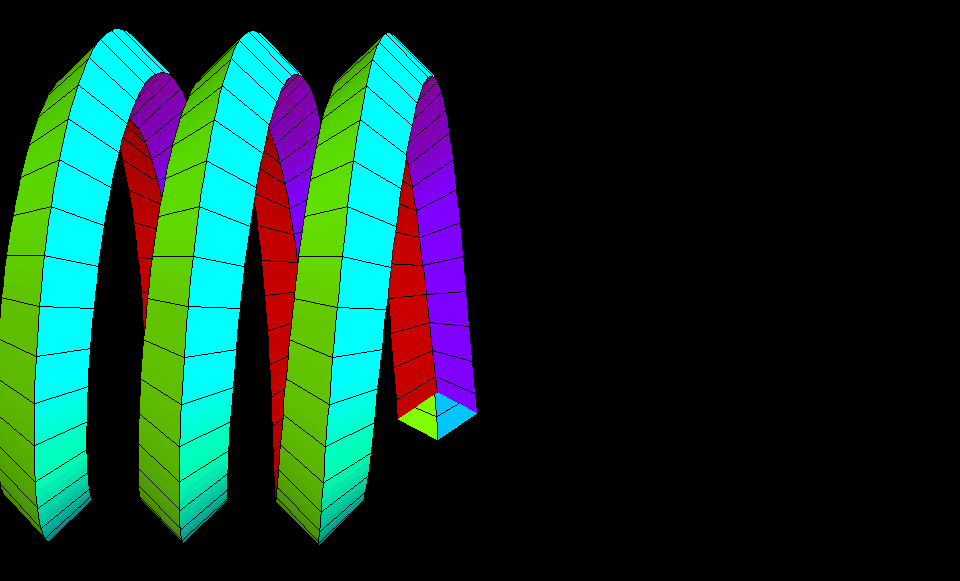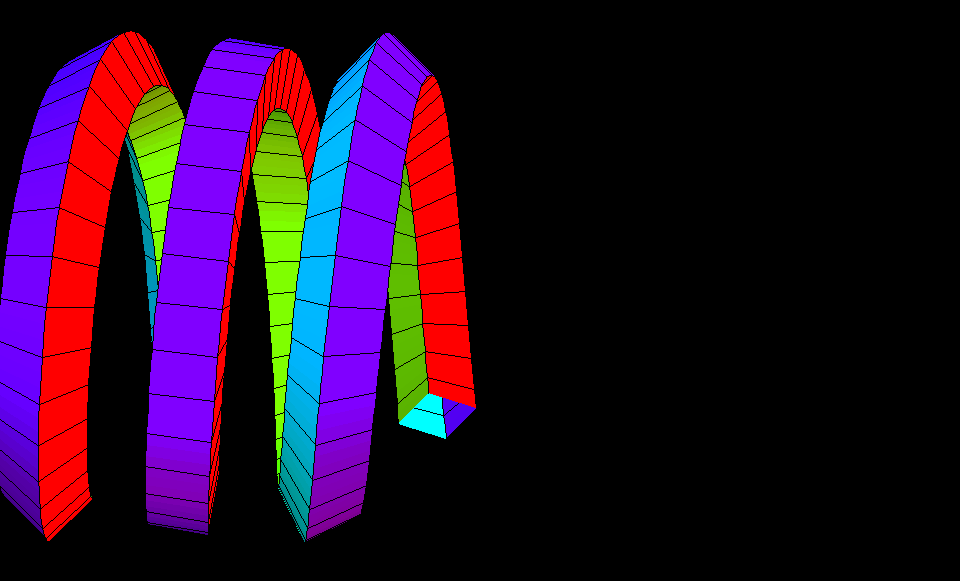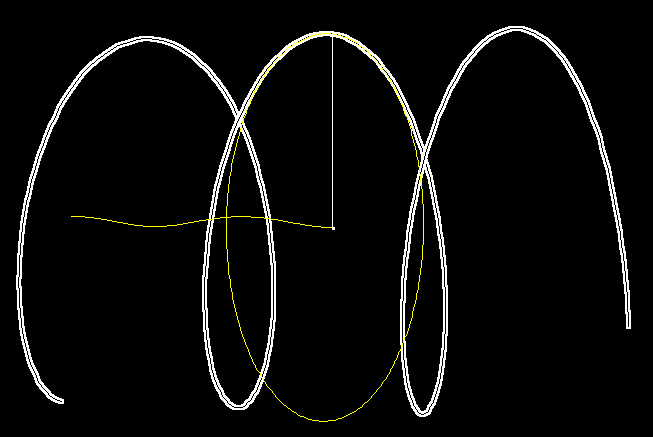# Helix

Back to interactive helicesA Circular Helix is a curve such that the angle of any tangent vector with the axix direction, is constant. A screw motion in the direction of the axis moves points along helices.

Alternatively, a Circular Helix is a space curve with constant curvature and constant torsion.Helix with "Frenet Tube" The edges of the tube are again helices. The inner edge (between red and purple) is determined by the curvature vector of the central helix. The curvature vector, at each point of the helix, points orthogonally to the axis.Helix as a square elastic tube. This tube behaves as an elastic metal spring (with square cross section of the wire) would behave. The edges of the tube wind around the helix curve. The animation shows that the cross section of the tube rotates faster when the helix is pulled to a longer helix. This twisting is well known to anyone who has “unwrapped” an electric coil by simply pulling it sideways. (watch the right end of the tube)Helix Circle Helix with curvature circle. After the circle the helix is the simplest curve with constant curvature and constant torsion. The curvature vector points from the helix towards the midpoint of the curvature circle and to the axis. As the curvature circle moves along the helix, the midpoints trace out another helix of the SAME constant curvature. The length of the curvature vector equals one over the radius of the curvature circle.

Helix.pdf